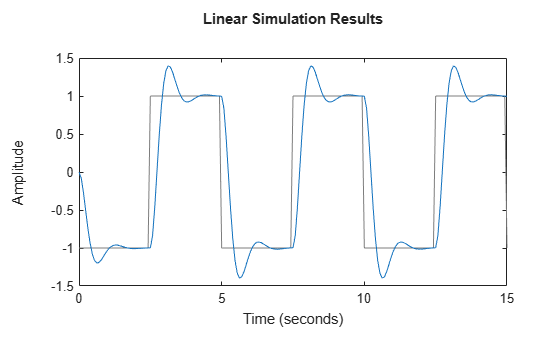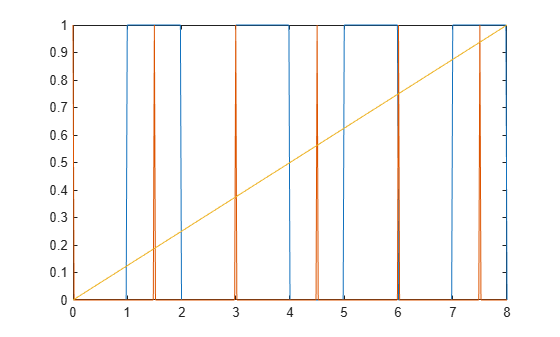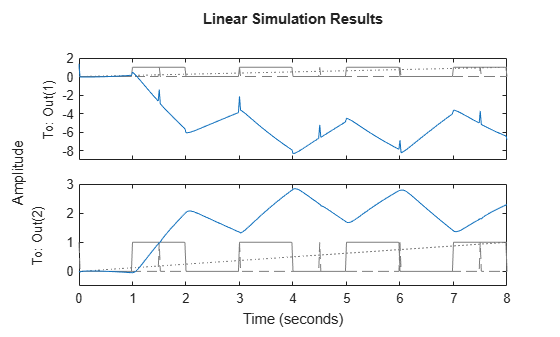gensig

Create periodic signals for simulating system response with lsim

Description

example

[u,t] = gensig(type,tau) generates a unit-amplitude periodic signal with the specified type and period. Use the signal u and corresponding time vector t to simulate the time response of a single-input dynamic system using lsim or lsimplot or to obtain response characteristics using lsiminfo. To create signals for multi-input systems, use repeated calls to gensig and stack the resulting u vectors into a matrix. When you use u and t to simulate a dynamic system model, the software interprets t as having the units of the TimeUnit property of the model.

example

[u,t] = gensig(type,tau,Tf) generates a signal with a duration of Tf. t runs from 0 to Tf in increments of tau/64.

example

[u,t] = gensig(type,tau,Tf,Ts) generates a signal with a sample time of Ts. t runs from 0 to Tf in increments of Ts. To generate a signal for simulating a discrete-time model, use this syntax and set Ts to the sample time of the model.

Examples

collapse all

Generate a square wave with a period of 2 s to use for simulating a dynamic system response with lsim.

tau = 2;
[u,t] = gensig("square",tau);

gensig returns the signal as the vector u and the corresponding time vector t. When you do not specify the duration of the signal, gensig generates a signal that runs for five periods (Tf = 5*tau). When you do not specify a time step, the function defaults to 64 samples per period (Ts = tau/64). Thus, this signal runs for 10 s with a time step of 0.03125 s. Plot the signal.

plot(t,u)gensig returns a square wave of unit amplitude that starts at zero. You can modify u to obtain a square wave with a different amplitude and different endpoints. Create a square wave of period 5 that runs for 15 s, and that switches between values of –1 and 1.

tau = 5;
Tf = 15;
[u0,t] = gensig("square",tau,Tf);
u = 2*u0-1;
plot(t,u)Use t and u to simulate the response of a dynamic system with lsim. The lsim command assumes the values of t are in the units of the dynamic system model that you simulate (sys.TimeUnit).

sys = tf(30,[1 5 30]);
lsim(sys,u,t)If you do not specify a time step (sample time), gensig defaults to 64 samples per period, or Ts = tau/64. When you want to simulate a discrete-time model with lsim, the time step must equal the sample time of the model. Provide gensig with this sample time to generate a suitable signal. For example, generate a sine wave for simulating a discrete-time dynamic system model with a 0.1 s sample time.

tau = 3;
Tf = 6;
Ts = 0.1;
[u,t] = gensig("sine",tau,Tf,Ts);

Simulate the model response to the generated signal.

sys = zpk([],[-0.1,-0.5],1,Ts);
lsim(sys,u,t,Ts)To simulate a multi-input system with lsim, you provide the input signals as a matrix whose columns represent the signal applied to each input. In other words, u(:,j) is the signal applied to the jth input at each time step. To use gensig to generate such an input matrix, create the signals for each input together and stack them together in a matrix.

For instance, create a signal for simulating a two-input system that injects a square wave of period 2 s into the first input, and a pulse every 1.5 s into the second input. Specify a duration and sample time so that the two vectors have the same length, which is necessary for combining them into a matrix.

Tf = 8;
Ts = 0.02;
[uSq,t] = gensig("square",2,Tf,Ts);
[uPu,~]  = gensig("pulse",1.5,Tf,Ts);
u = [uSq uPu];
size(u)
ans = 1×2

401     2

Each row u(i,:) of u is the signal (u1,u2) applied to the inputs at the corresponding time t(i).

You can combine signals that are not created with gensig provided they have the same length. For instance, suppose that you want to simulate a three-input system by applying uSq to the first input and uPu to second input. You also want to apply a ramp to the third input that starts at 0 and increases to 1 at the final time Tf = 8. Ensure that the signal is a column vector with the same length as uSq and uPu. Then combine it with the other signals to create the input matrix.

uRa = linspace(0,1,401)';
u = [uSq uPu uRa];
size(u)
ans = 1×2

401     3

plot(t,u)You can now use u and t to simulate a three-input model. Generate a three-input, two-output state-space model, and simulate the response at its two outputs to u applied at the inputs.

rng('default')
lsim(sys,u,t)Input Arguments

collapse all

Type of periodic signal to generate, specified as one of the following:

• "sine" — Sine wave

• "square" — Square wave

• "pulse" — Periodic pulse

All signals have unit amplitude and have the initial value 0 at t = 0. You can specify the type with a character vector instead of a string (for example, 'sine').

Period of generated signal, specified as a positive scalar value. Specify tau in the units of the dynamic system model you want to simulate with lsim. For instance, if sys.TimeUnit is 'seconds', then to generate a signal for simulating sys with a period of 30 s, set tau to 30. If sys.TimeUnit is 'minutes', then to generate such a signal, set tau to 0.5.

Duration of signal for simulation, specified as a positive scalar value. The output vector t is of the form 0:Ts:Tf, where the time step is set by Ts. When you use t with lsim to simulate a dynamic system model, lsim interprets t as having the units specified in the TimeUnit property of the model.

Time step, specified as a positive scalar value. The output vector t is of the form 0:Ts:Tf. The units of Ts are the units specified by the TimeUnit property dynamic system model you intend to simulate with lsim. When you are simulating a discrete-time model, set Ts equal to the sample time Ts of the model.

Output Arguments

collapse all

Generated signal, returned as a column vector of the same length as t. The shape of the signal is determined by type. The signal has unit amplitude with a baseline of 0.

Time vector, returned as a column vector of the form 0:Ts:Tf. If you do not specify a duration Tf, then gensig uses Tf = 5*tau. If you do not specify a time step Ts, then gensig uses tau/64.